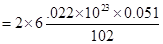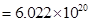## शनिवार, 29 जुलाई 2017

### ATOMS AND MOLECULES PART-II

Chapter – end)
1.A 0.24 g sample of compound of oxygen and boron was found by analysis to contain 0.096 g of boron and 0.144 g of oxygen. Calculate the percentage composition of the compound by weight.
Ans. Mass of the given sample compound = 0.24g
Mass of boron in the given sample compound = 0.096g
Mass of oxygen in the given sample compound = 0.144g
% composition of compound = % of boron and % of oxygen
Therefore % of boron = mass of boron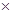100/mass of the sample compound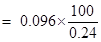= 40%
Therefore % of oxygen = mass of oxygen100/mass of the sample compound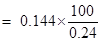= 60%

2.When 3.0 g of carbon is burnt in 8.00 g oxygen, 11.00 g of carbon dioxide is produced. What mass of carbon dioxide will be formed when 3.00 g of carbon is burnt in 50.00 g of oxygen? Which law of chemical combination will govern your answer?
Ans. According to the law of chemical combination of constant proportions “in a chemical compound the elementary constituents always combine in constant proportions by weight/mass”. Therefore, whether 3 g carbon is burnt in 8 g oxygen or 3g carbon is burnt in 50g oxygen in both cases only 11g carbon dioxide will be formed.

3.What are polyatomic ions? Give examples.
Ans. When two or more atoms in a group is having a charge, such is called a polyatomic ion. For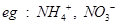etc.

4.Write the chemical formulae of the following.
(a) Magnesium chloride
(b) Calcium oxide
(c) Copper nitrate
(d) Aluminium chloride
(e) Calcium carbonate.
Ans.5.Give the names of the elements present in the following compounds.
(a) Quick lime
(b) Hydrogen bromide
(c) Baking powder
(d) Potassium sulphate.
Ans.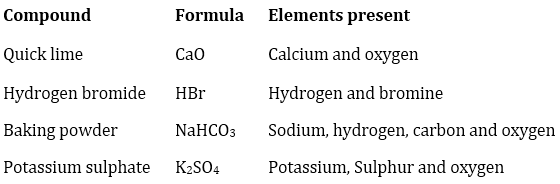6. Calculate the molar mass of the following substances.
(a) Ethyne,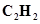(b) Sulphur molecule,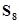(c) Phosphorus molecule,(Atomic mass of phosphorus= 31)
(d) Hydrochloric acid, HCl
(e) Nitric acid,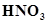Ans. (a)Ethyene =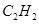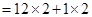=24 + 2= 26 u =26 g
(b)Sulphur molecular =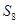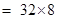= 256 u= 256 g
(c)Phosphorus molecule =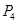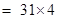= 124 u= 124 g
(d)Hydrochloric acid = HCl = 1+ 35.5 = 36.5 u= 36.5 g
(e)Nitric acid =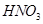= 1 + 14 +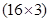= 15 + 48 = 63 u =63 g

7.What is the mass of—
(a) 1 mole of nitrogen atoms?
(b) 4 moles of aluminium atoms (Atomic mass of aluminium= 27)?
(c) 10 moles of sodium sulphite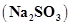?
Ans. (a)Atomic mass of nitrogen is 14 u.
therefore 1 mol of N = 14g
(b)Atomic mass of aluminium = 27u
therefore 1 mol of Al = 27g and so 4 mol of Al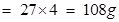(c)molecular mass of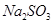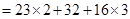= 46 + 32 + 48 = 126 u
therefore 1 mol ofhas weight/mass 126g.
hence, 10 mol of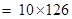= 1260g

8.Convert into mole.
(a) 12 g of oxygen gas
(b) 20 g of water
(c) 22 g of carbon dioxide.
Ans. (a)molecular mass of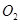= 32 u= 32g(1 mole)
since 32 g of=1mole then 12g of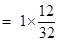=0.375mole.
(b)molecular mass of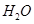+ 16 = 18 u= 18g(1mole)
20g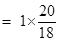= 1.11mole.
(c)molecular mass of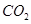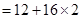= 12 + 32 = 44 u= 44g (1mole)
22g of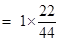= 0.5mole.

9.What is the mass of:
(a) 0.2 mole of oxygen atoms?
(b) 0.5 mole of water molecules?
Ans. (a) since 1 mole of O = atomic mass of O = 16u=16g
then 0.2mole of(b)1mol of H2O = molecular mass of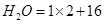=18 u =18g
then 0.5mol of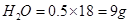10.Calculate the number of molecules of sulphur (S8) present in 16 g of solid sulphur.
Ans. 1mol of= molecular mass of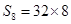= 256u=256g
since 256g of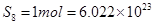atoms (Avogadro number)
16g of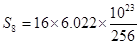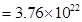molecules

11.Calculate the number of aluminium ions present in 0.051 g of aluminium oxide.
(Hint: The mass of an ion is the same as that of an atom of the same element. Atomic mass of Al = 27 u)
Ans. 1mol of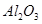= molecular mass of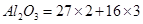= 102u=102g
aluminium ions present in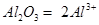102 g ofcontains aluminium ions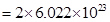then 0.051 gcontains aluminium ions